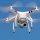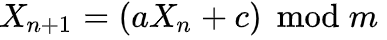Home
IT Knowledge
Inspiration
Languages
EN

# JavaScript - own random number generator (custom implementation)

11 points
Created by:Marcin
19550

In this article, we're going to have a look at how to write own random numbers genrator that is based on LCG (Linear congruential generator) algorithm in JavaScript.

## 1. Introduction

In JavaScript we can implement custom random number generator by using LCG (Linear congruential generator) algorithm. LCG is one of the oldest and best-known pseudorandom number generator algorithm. We can adjust this implementation to work on int or long.

Linear congruential generator is defined by recurrence relation:Where:

• `Xn+1` - new seed
• `a` - MULTIPLIER_A
• `Xn` - current seed field
• `c` - INCREMENT_C
• `m` - MODULUS

## 2. Implementation

Note: every time `randomInt()` method is called, it is necessary to update seed value.

``````// ONLINE-RUNNER:browser;

function RandomUtil(seed) {
var self = this;

if (seed == null) {
seed = 3819201;
}

var MULTIPLIER_A = 1103515245;
var INCREMENT_C = 12345;
var MODULUS = 2147483647; // max integer value

self.randomInt = function() {
seed = (seed * MULTIPLIER_A + INCREMENT_C) % MODULUS;
return seed;
};
}

// Usage example 1:

var util = new RandomUtil();

console.log(util.randomInt()); // 348328093
console.log(util.randomInt()); // 121065958
console.log(util.randomInt()); // 790325445

// Usage example 2:

var now = new Date();
var util = new RandomUtil(now.getTime());

// Values should be different always:
console.log(util.randomInt()); // 922892575
console.log(util.randomInt()); // 1117277734
console.log(util.randomInt()); // 2084933141``````